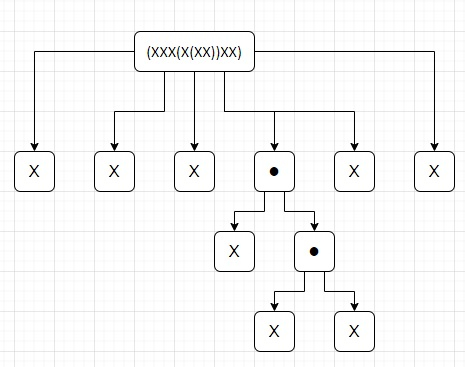# Program to count number of characters in each bracket depth in Python

PythonServer Side ProgrammingProgramming

Suppose we have a string s which consists of only three characters "X", "(", and ")". The string has balanced brackets and in between some "X"s are there along with possibly nested brackets may also there recursively. We have to find the number of "X"s at each depth of brackets in s, starting from the shallowest depth to the deepest depth.

So, if the input is like s = "(XXX(X(XX))XX)", then the output will be [5, 1, 2]To solve this, we will follow these steps −

• depth := -1
• out := a new list
• for each c in s, do
• if c is same as "(", then
• depth := depth + 1
• otherwise when c is same as ")", then
• depth := depth - 1
• if depth is same as size of out , then
• insert 0 at the end of out
• if c is same as "X", then
• out[depth] := out[depth] + 1
• return out

## Example

Let us see the following implementation to get better understanding −

def solve(s):
depth = -1
out = []

for c in s:
if c == "(":
depth += 1
elif c == ")":
depth -= 1

if depth == len(out):
out.append(0)

if c == "X":
out[depth] += 1
return out

s = "(XXX(X(XX))XX)"
print(solve(s))

## Input

"(XXX(X(XX))XX)"

## Output

[5, 1, 2]